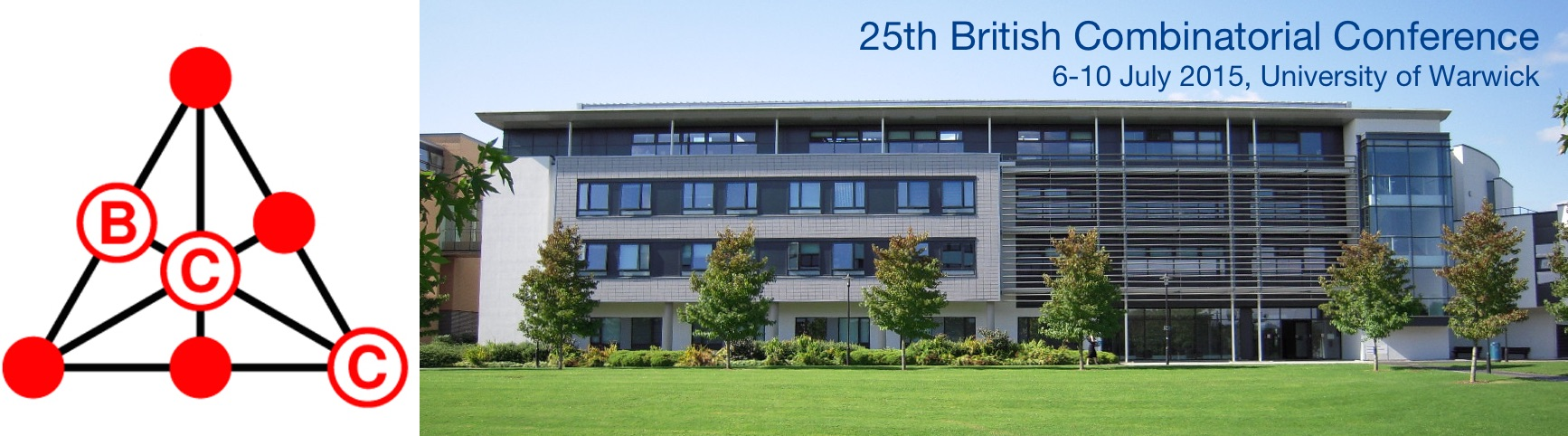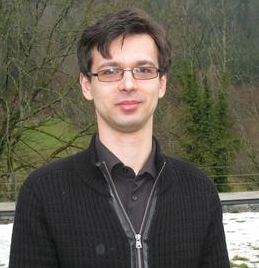# Invited speakers## Invited speakersManuel Bodirsky
TU, Dresden

#### Ramsey Classes: Examples and Constructions

This work is concerned with classes of relational structures that are closed under taking substructures and isomorphism, that have the joint embedding property, and that furthermore have the Ramsey property, a strong combinatorial property which resembles the statement of Ramsey's classic theorem. Such classes of structures have been called Ramsey classes. Nešetřil and Rödl showed that they have the amalgamation property, and therefore each such class has a homogeneous Fraïssé limit. Ramsey classes have recently attracted attention due to a surprising link with the notion of extreme amenability from topological dynamics. Other applications of Ramsey classes include reduct classification of homogeneous structures.

We give a survey of the various fundamental Ramsey classes and their (often tricky) combinatorial proofs, and about various methods to derive new Ramsey classes from known Ramsey classes. Finally, we state open problems related to a potential classification of Ramsey classes.David Conlon
University of Oxford

#### Recent developments in graph Ramsey theory

Given a graph H, the Ramsey number r(H) is the smallest natural number N such that any two-colouring of the edges of KN contains a monochromatic copy of H. The existence of these numbers has been known since 1930 but their quantitative behaviour is still not well understood. Even so, there has been a great deal of recent progress on the study of Ramsey numbers and their variants, spurred on by the many advances across extremal combinatorics. In this survey, we will describe some of this progress.Stefanie Gerke
Royal Holloway,
University of London

#### Controllability and matchings in random bipartite graphs

Motivated by an application in controllability we consider maximum matchings in random bipartite graphs G=(A,B). First we analyse Karp-Sipser's algorithm to determine the asymptotic size of maximum matchings in random bipartite graphs with a fixed degree distribution. We then allow an adversary to delete one edge adjacent to every vertex in A in the more restricted model where each vertex in A chooses d neighbours uniformly at random from B.Gil Kalai
Hebrew University of Jerusalem

#### Some old and new problems in combinatorial geometry I: Around Borsuk's problem

Borsuk asked in 1933 if every set of diameter 1 in Rd can be covered by d+1 sets of smaller diameter. In 1993, a negative solution, based on a theorem by Frankl and Wilson, was given by Kahn and Kalai. In this work I will present questions related to Borsuk's problem.Tomasz Łuczak

#### Randomly generated groups

We discuss some older and a few recent results related to randomly generated groups. Although most of them are of topological and geometric flavour the main aim of this work is to present them in combinatorial settings.Gary McGuire
University College Dublin

#### Curves over finite fields and linear recurring sequences

We investigate what happens when we apply the theory of linear recurring sequences to certain sequences that arise from curves over finite fields. The sequences we will study are an:= #C(Fqn)- (qn+1) where #C(Fqn) is the number of Fqn-rational points on a curve C defined over Fq.Sergey Norin
McGill University, Montreal

#### New tools and results in graph minor structure theory

Graph minor theory of Robertson and Seymour is a far reaching generalization of the classical Kuratowski-Wagner theorem, which characterizes planar graphs in terms of forbidden minors. We survey new structural tools and results in the theory, concentrating on the structure of large t-connected graphs, which do not contain the complete graph Kt as a minor.Nik Ruškuc
University of St Andrews, Scotland

#### Well quasi-order in combinatorics: embeddings and homomorphisms

The notion of well quasi-order (wqo) from the theory of ordered sets often arises naturally in contexts where one deals with infinite collections of structures which can somehow be compared, and it then represents a useful discriminator between 'tame' and 'wild' such classes. In this work we survey such situations within combinatorics, and attempt to identify promising directions for further research. We argue that these are intimately linked with a more systematic and detailed study of homomorphisms in combinatorics.Chaoping Xing
Nanyang Technological University

#### Optimal rate algebraic list decoding of folded algebraic geometry codes

We give a construction of folded algebraic geometry codes which are list decodable from a fraction 1-R-ε of adversarial errors, where R is the rate of the code, for any desired positive constant ε.

By using explicit towers, we obtain folded algebraic geometry code with the worst-case list size output O(1/ε), matching the existential bound for random codes up to constant factors. Further, the alphabet size of the codes is a constant depending only on ε – it can be made exp(O(1/ε2)) which is not much worse than the lower bound of exp(Ω(1/ε)). The parameters we achieve are thus quite close to the existential bounds in all three aspects – error-correction radius, alphabet size, and list-size – simultaneously. Our code construction is Monte Carlo and has the claimed list decoding property with high probability. Once the code is (efficiently) sampled, the encoding/decoding algorithms are deterministic with a running time Oε (Nc) for an absolute constant c, where N is the code's block length.

By using class field towers, we obtain folded algebraic geometry code with the worst-case list size output O(poly(N)). The alphabet size of the codes is a constant depending only on ε. Our code construction is deterministic. Once the code is efficiently encoded, decoding algorithms are efficiently as well.